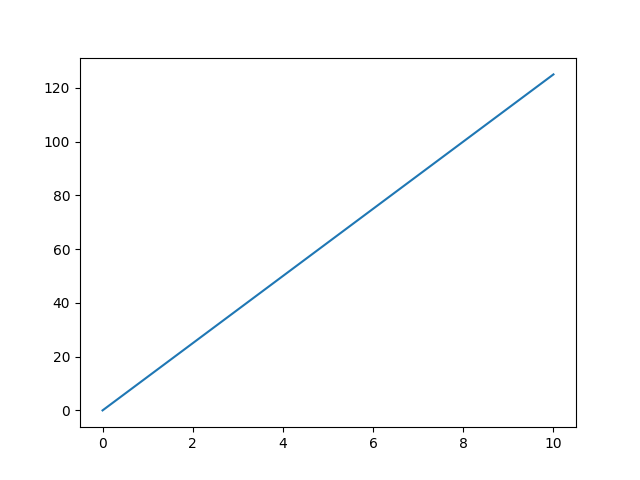## 31 Jan Matplotlib – PyPlot

The PyPlot is a sub module and  a lot of Matplotlib functionalities reside in it, therefore import it as shown below:

The following are the methods you can use to create different plots with Matplotlib sub module PyPlot:

• bar(): For a bar plot
• hist(): Plot a Histogram
• pie(): Plot a pie chart
• scatter(): Form a scatter plot
• stem(): Form a stem plot
• step(): Form a step plot

Let us now see an example wherein we will use the Matplotlib module PyPlot and draw:

OutputIf you liked the tutorial, spread the word and share the link and our website Studyopedia with others.